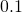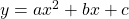Let’s plot the points on the graph that satisfy. That is, for each value ofplot.

The integer values are easy to consider. By plotting points at increments ofwe see the form of a parabola.

Every quadratic function of the formhas this same shape. However, the coefficientsandalter the position and steepness of the parabola.

Use the sliders below to create a variety of parabolas. Try this identify parabola task.